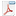# Popularity bigdata / large data packages in R and ffbase useR presentation

A few weeks ago, Rstudio released it's download logs, showing who downloaded R packages through their CRAN mirror. More info: http://blog.rstudio.org/2013/06/10/rstudio-cran-mirror/

This is very nice information and it can be used to show the popularity of packages with R, which has been done before and criticized also as the RStudio logs might/might not be representative for the download behaviour of all useRs.As the useR2013 conference has come to an end, one of the topics corporate useRs of R seem to be talking about is how to speed up R and how R handles large data.
Edwin & BNOSAC did their fair share by giving a presentation about the use of ffbase alongside the ff package which can be found here.

When looking at twitter feeds (https://twitter.com/search?q=user2013), there is now Tibco who has it's own R interpreter, there is R inside the JVM, Rcpp, Revolution R, ff/ffbase, R inside Oracle, there is pbdR, pretty quick R (pqR), MPI, R on grids, R with mongo/monet-DB, PL/R, dplyr and useRs made a lot of presentations about how they handled large data in their business setting. It seems like the use of R with large datasets is being more and more accepted in the corporate world - which is a good thing. And we love the diversity!

For R packages which are on CRAN, the Rstudio download logs can be used to show download statistics of the open source bigdata / large data packages which are now on the market (CRAN).
For this, the logs were downloaded and a number of open source packages which are out-of-memory / bigdata solutions in R were compared with respect to download stats on this mirror.

It seems like by far the most popular package is ff and our own contribution (ffbase) is not doing bad at all (+/- 100 ip addresses downloaded our package per week from the Rstudio CRAN mirror only).

If you are interested in the code to download the data and get the plot or if you want to compare your own packages, you can use the following code.

```##
## Rstudio logs
##
input <- list()
input\$path <- getwd()
input\$path <- "/home/janw/Desktop/ffbaseusage"
input\$start <- as.Date('2012-10-01')
input\$today <- as.Date('2013-06-10')
input\$today <- Sys.Date()-1
input\$all_days <- seq(input\$start, input\$today, by = 'day')
input\$all_days <- seq(input\$start, input\$today, by = 'day')
input\$urls <- paste0('http://cran-logs.rstudio.com/',
as.POSIXlt(input\$all_days)\$year + 1900, '/', input\$all_days, '.csv.gz')
##
##
sapply(input\$urls, FUN=function(x, path) {
print(x)
}, path=input\$path)

##
## Import the data in a csv and put it in 1 ffdf
##
require(ffbase)
files <- sort(list.files(input\$path, pattern = ".csv.gz\$"))
rstudiologs <- NULL
for(file in files){
print(file)
con <- gzfile(file.path(input\$path, file))
x <- read.csv(con, header=TRUE, colClasses = c("Date","character","integer", rep("factor", 6), "numeric"))
x\$time <- as.POSIXct(strptime(sprintf("%s %s", x\$date, x\$time), "%Y-%m-%d %H:%M:%S"))
rstudiologs <- rbind(rstudiologs, as.ffdf(x))
}
dim(rstudiologs)
rstudiologs <- subset(rstudiologs, as.Date(time) >= as.Date("2012-12-31"))
ffsave(rstudiologs, file = file.path(input\$path, "rstudiologs"))

library(ffbase)
library(data.table)
tmp <- ffload(file.path(input\$path, "rstudiologs"), rootpath = tempdir())
rstudiologs[1:2, ]
packages <- c("ff","ffbase","bigmemory","mmap","filehash","pbdBASE","colbycol","MonetDB.R")
idx <- rstudiologs\$package %in% ff(factor(packages))
idx <- ffwhich(idx, idx == TRUE)
mypackages <- rstudiologs[idx, ]
mypackages <- as.data.frame(mypackages)
info <- c("r_version","r_arch","r_os","package","version","country")
mypackages[info] <- apply(mypackages[info], MARGIN=2, as.character)
mypackages <- as.data.table(mypackages)
mypackages\$aantal <- 1
mondayofweek <- function(x){
weekday <- as.integer(format(x, "%w"))
as.Date(ifelse(weekday == 0, x-6, x-(weekday-1)), origin=Sys.Date()-as.integer(Sys.Date()))
}
mypackages\$date <- mondayofweek(mypackages\$date)
byday <- mypackages[,
list(aantal = sum(aantal),
ips = length(unique(ip_id))),
by = list(package, date)]
byday <- subset(byday, date != max(as.character(byday\$date)))

library(ggplot2)
byday <- transform(byday, package=reorder(package, byday\$ips))
qplot( data=byday, y=ips, x=date, color=reorder(package, -ips, mean), geom="line", size=I(1)
) + labs(x="", y="# unique ip", title="Rstudio logs 2013, downloads/week", color="") + theme_bw()

```